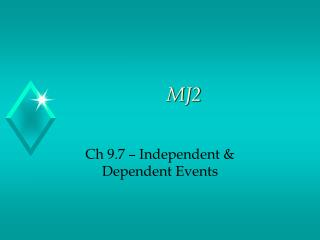DownloadDownload PresentationMJ2

# MJ2

Télécharger la présentation## MJ2

- - - - - - - - - - - - - - - - - - - - - - - - - - - E N D - - - - - - - - - - - - - - - - - - - - - - - - - - -
##### Presentation Transcript

1. MJ2 Ch 9.7 – Independent & Dependent Events

2. Bellwork • Between 3:00 P.M. and 4:00 P.M., 11 sedans, 15 trucks, and 6 sports cars drove through an intersection. Based upon this information, what is the probability that a vehicle that drives through the intersection is a sports car? Note: You do not have to write the question

3. Assignment Review • Text p. 395 # 6 – 13

4. Before we begin… • Please take out your notebook and get ready to work… • In yesterday’s lesson we worked with experimental and theoretical probability… • In today’s lesson we will look at scenarios in which the outcome of one event may or may not affect the outcome of another event

5. Objective • Students will find the probability of independent and dependent events

6. Vocabulary • Compound Event – A compound event consists of two or more simple events • Independent Events – The outcome of one event does not affect the other event. • Dependent Events – The outcome of one event affects the outcome of another event • Let’s look at independent events first…

7. Independent Events • The probability of two independent events can be found by multiplying the probability of the first event by the probability of the second event. This can be written with the following formula P (A and B) = P(A) ∙ P(B) • Let’s look at an example…

8. P (independent events) • The two spinners are spun. What is the probability that both spinners will show and even number? P(1st spinner even) = 4 8 P(2nd spinner even) = 4 8 P(both spinners even) =4 ∙ 4 = 16 = 1 8 8 64 4

9. Your Turn • A game uses a number cube and a spinner. • A player rolls a number cube. What is the P(odd #)? • The player spins the spinner. What is the P(red)? • What is the P(odd # and Red)?

10. Dependent Events • If two events A and B, are dependent, then the probability of both events occurring is the product of the probability of A and the probability of B, after A occurs. You can use the following formula to represent this probability P(A and B) = P(A) ∙ P(B after A) • In plain English this means that after event A occurs you reduce the # of favorable outcomes and the # of total outcomes • Let’s look at an example…

11. Example • There are 4 red, 8 yellow and 6 blue socks in a drawer. Once a sock is selected it is not replaced. Find the probability that 2 blue socks are chosen. P(1st blue sock) = 6 18 P(2nd blue sock) = 5 17 P(Two blue socks) = 6 ∙ 5 = 30 = 5 18 17 306 51 # of socks after 1 blue is removed Total # of socks after 1 blue is removed

12. Your Turn • In the notes section of your notebook write a probability statement and determine the probability • There are 3 yellow, 5 red, 4 blue and 8 green candies in a bag. Once a candy is removed it is not replaced. Find the probability: • P(two red candies) • P(two blue candies) • P(yellow candy followed by a blue candy)

13. Summary • In the notes section of your notebook summarize the key concepts covered in today’s lesson. • Today we discussed • Probability of compound events • What are compound events • What are independent and dependent events?

14. Assignment • Text p. 400 # 6 – 16 Reminder • This assignment is due tomorrow • I do not accept late assignments • I do not accept answers only for any assignment